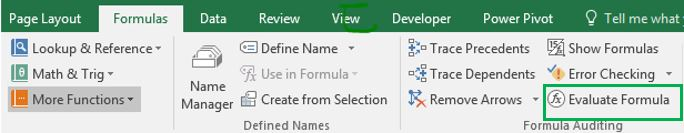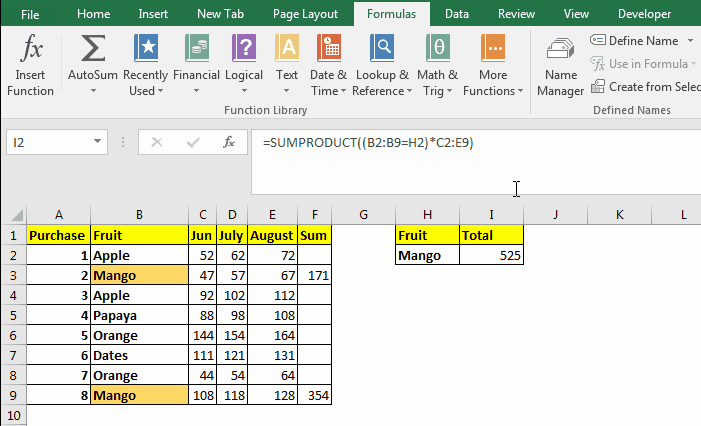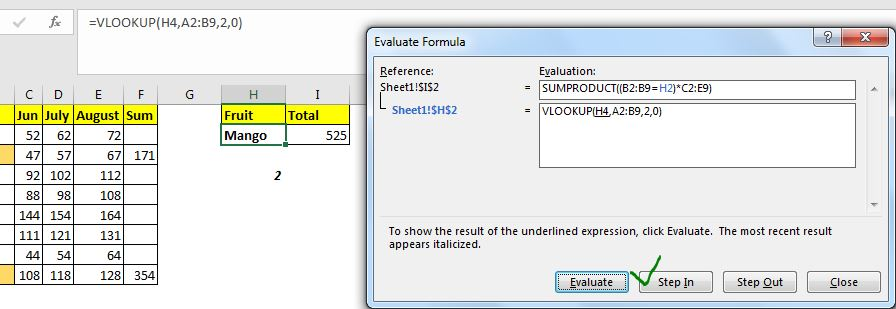# Evaluation of Excel Formula Step By Step

Excel provides functionality that helps us evaluate the formula. We can see how a formula is working by stepping through the formula.  In this article, we will learn how to use evaluate the formula option of Excel.So, in a recent article, we learned how to sum multiple columns on one condition. Let's try to know how that formula is being evaluated.

### Evaluate formula using excel evaluate formula option

So the formula was:

 =SUMPRODUCT((B2:B9=H2)*C2:E9)

Let's evaluate it.Select the cell that contains the formula and:

• Go to --> Menu--> Formula--> Formula Auditing group--> Evaluate Formula.
• Now click on Evaluate. It will first solve all the underlined part of the formula. You can see the underlined part in the box. The underlined part is currently under evaluation. The Evaluate method usually solves references in parenthesis first (follows the BODMAS).
• Click again on the Evaluate button. This time it solves the comparison expression and returns true false (see the above gif).
• On the next click, it opens the parenthesis.
• Clicking again shows the result of the multiplication of the two arrays.
• Finally, it shows the result returned by the SUMPRODUCT function and Evaluate Button turns into the Restart button.

The above steps were for the formula used in a specific problem. The procedure changes with changing formulas. Now there were two more buttons. the Step In and Step Out button. Let's see what the do.

### The Step In and Step Out FormulaThe Step In button is used when a formula depends on another formula. To evaluate that formula too, we use the Step in button.

For example, in the above image, the excel formula refers to H2 and H2 itself contains a formula. So, when we click on the Evaluate option, we see the step In button usable. If you click on the Step in button, it will show the formula H2 contains. Now if H2 refers to a cell that itself contains a formula, you will still see Step in option available.

When you click on the Step out button, it will insert the values returned by those references in the original formula. And finally, we start clicking on the Evaluate button to see how it working.

Using Evaluate Formula Method to Debug Errors:

I mostly use it when I am trying to figure out why the formula is resulting in an errorful output. I use it to debug and trace the error source. It can help you find the mistakes you've done while writing this formula.

So yeah guys, this how you can use the Evaluate Formula method to see step by step calculation excel does in the background. I hope I was explanatory enough. If you have any doubts regarding this or any other excel/VBA related query, ask in the comments section below.

Related Articles:

F9 Key to Debug Excel Formulas | The F9 key is very useful to debug excel formula in bits and parts. To see what a specific part of the formula returns we can use the F9 key. Just select that part of the formula and hit F9.

How to Trace and Fix Formula Errors in Excel | The when a formula that refers to multiple cells and ranges in excel sheet, it gets hard to locate the error. The option Trace Error in Excel helps a lot.

Formula Errors in Excel and Solutions | There are many types of errors that occur in excel. It is good to know which error occurs when in excel. Each error indicates the reason of the error and they have their specific solution in Excel.

Popular Articles:

50 Excel Shortcuts to Increase Your Productivity | Get faster at your task. These 50 shortcuts will make your work even faster on Excel.

The VLOOKUP Function in Excel | This is one of the most used and popular functions of excel that is used to lookup value from different ranges and sheets.

COUNTIF in Excel 2016 | Count values with conditions using this amazing function. You don't need filter your data to count specific value. Countif function is essential to prepare your dashboard.

How to Use the SUMIF Function in Excel | This is another dashboard essential function. This helps you sum up values on specific conditions.

Terms and Conditions of use

The applications/code on this site are distributed as is and without warranties or liability. In no event shall the owner of the copyrights, or the authors of the applications/code be liable for any loss of profit, any problems or any damage resulting from the use or evaluation of the applications/code.